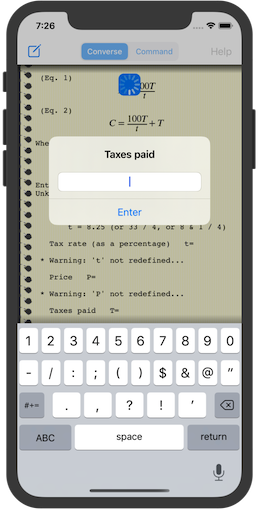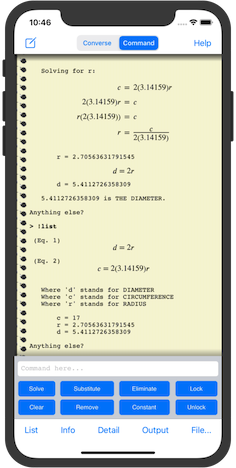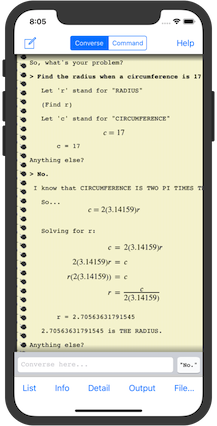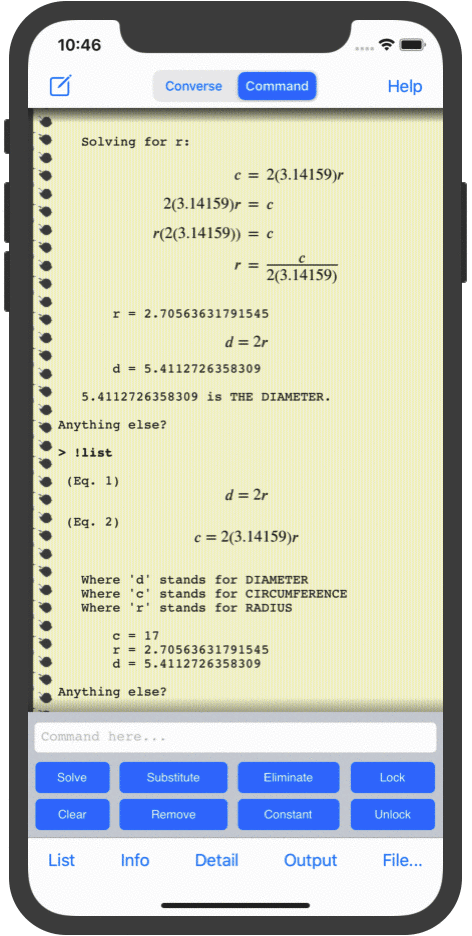# AutoMathicThe Smart Calculator for Automated Math

(C) 1988, 2021  Kevin B. Belton

 Home Features Screenshots Examples Testimonials News Documentation Support

Global Features
• Its output scrolls indefinitely, showing variables, expressions, and equations that are formatted and easy to read!
• It automates most of the problem-solving process (even when in the hands-on "Command" mode)!
• It has customizable level-of-detail settings that can show and explain every step of the way!
• Variables, equations, and unknowns can be listed at any time so you always know the current state!
• Transcripts can be saved to record and document the entire session!  You can even share or export transcripts with the iOS Files app or iTunes!
• The Algebra (equations and variables) can be saved and reused to perform similar calculations without setting them up!  Algebra files can be shared with the iOS Files app or iTunes!

Mode-Specific Features

"Scripted" mode "Command" mode "Converse" mode
AutoMathic lets you run scripted calculations from a large, built-in library if you want to do something commonplace.  Scripted calculations are pre-built workflows that set up and perform complete calculations automatically! AutoMathic lets you do manual calculations by accepting expressions and equations you enter yourself, and gives you the tools to manipulate them using familiar techniques from algebra. AutoMathic lets you do free-form calculations by translating English-like, conversational input into variables, expressions, and equations to be solved...  and then it solves them!  You tell it what you know, and ask it what you want to find out!Instead of rigid equation libraries, it has a collection of task-oriented workflows that conform to how you want to use them!

• They often include comments, equations, expressions, variables, interactive input, and other Command-mode commands.

• Pop-up prompts / instructions guide you to provide input.

• They don't even distinguish between variables for "input" and variables for "output"!

• Input can be any valid expression, made up of numbers, operators, and/or variables.

• Unless otherwise instructed, entering nothing will treat the value being asked for as an unknown.

• Unknowns will be found automatically if possible.

• Additional or follow-up calculations can be done manually from Command mode.

AutoMathic includes a library of scripted calculation files that use all of the Command-mode features to perform many useful, task-oriented calculations:

• Basic Pricing
• Complex Pricing
• Meal Calculator
• Fuel Consumption
• Percentages
• Percent Change
• Simple Interest

• Unit conversion:

• Microscopic Units
• Macroscopic Units
• Astronomical Units
• Weight / Mass Units
• Liquid Volume Units
• Temperature Units
• Pressure Units
• Angular Measurement Units

• Elementary algebra:

• Arithmetic Sequences
• Integer Summation
• Circles
• Squares
• Rectangles
• Trapezoids
• Triangles
• Equilateral Triangles
• Isosceles Triangles
• Right Triangles
• Interpolation
• Equations of a Line
• Midpoint of a Line
• Intersecting Lines
• Parallel Lines
• Perpendicular Lines

• Elementary statistics:

• Simple Average
• Weighted Average
• Basic Probability
• Z-scores
• Skewness

• Elementary physics:

• Linear Velocity
• Angular Velocity

• Elementary chemistry:

• Atomic Properties

You can create your own custom scripts and import them into AutoMathic using the iOS Files app or iTunes File Sharing!
Instead of requiring "programming" with a low-level programming language, it lets you create, use, save, and later reuse your own equations just like the built-in equation libraries!

Command mode gives you direct, low-level control to perform the following problem-solving tasks using the graphical user interface, or using the Command line itself:

• Evaluating expressions

• Storing equations for evaluation

• Solving equations for a variable

• Rewriting equations to substitute variables

• Rewriting equations to eliminate variables

• Turning variables into numeric constants

• Turning variables into symbolic constants

• Erasing variables

• Erasing equationsButton Action Solve Solve an equation for a particular variable Substitute Replace a variable symbolically in all equations Eliminate Alter equations to omit references to a variable Constant Replace a variable with its current numeric value Lock Prevent variables from changing values Unlock Turn locked variables back into regular variables Clear Undefine any or all evaluated variables Remove Erase one or all stored equations

AutoMathic runs on the iPhone, iPod touch, and iPad!

Version 2.3.1
Available Now!

Converse mode has more optimizations to its indirect-solution strategies, new vocabulary supporting "numeric fraction words" (e.g. "3rds", "10ths", "16ths", "100ths"), and vocabulary standardizations for consistent unit translation!AutoMathic offers a high-level, "natural-language" interface that accepts free-form, conversational input and automatically pulls in whatever background information is needed at the time from a broad, built-in knowledgebase!You can even use your voice to enter conversational input as speech! *

Basic Behavior and Language Skills

• Accepts free-form input in a restricted subset of the English language.

• Automatically selects variables for nouns and noun phrases.

• Automatically matches singular and plural forms of words, including alternate plural forms.

• Fuzzy-matches noun phrases to determine if two or more noun phrases refer to the "same thing".

• Understands the sometimes-special meaning of quantity words such as "number", "amount", "fraction", "quantity", "part", "portion", "multiple", and "percentage".

• Automatically generates appropriate variable names for implicit "how much", "how many", and "what" questions.

• Allows use of any demonstrative pronoun (this, that, these, those, them) for referring to the most recent noun or answer given.

• Handles algebraic math expressions using the symbols "+", "-", "*", "x", "/", "=", "(", ")", and "%".

• Handles arbitrary units, using a few language conventions.

• Handles all common forms and tenses of the verb "to be", alone or in combination with the auxiliary verb forms of "have" and "do", and modal auxiliary verbs such as "should", "would", "could", "must", etc.

• Rejects translations which are not interpreted as valid equations or expressions.

Vocabulary and Knowledgebase

• Recognizes simple numbers and fractions by name (e.g. one, dozen, fifteen, ninety, five billion, three tenths, five sixteenths, 3 10ths, 5/16ths).

• Knows a few special constants by their common names (e.g. Pi, Tau, e, Golden Ratio, C).

• Knows some common factors by name (e.g. half, double, triple, quadruple).

• Knows standard abbreviations for many simple and compound units that have unique and unambiguous abbreviations (e.g. cm, km, qt, oz, mph, rpm, cc's).

• Consults an extensive library of mathematical facts as needed, and incrementally loads only the relevant information.

Indirect Solution Strategies

When AutoMathic cannot solve a problem by plugging-in the values of known variables to find unknowns, it may try to find them using a few indirect strategies:

1. Create a hypothetical situation by assigning a value to a variable.

2. Make opportunistic substitution(s) which simplify a system of equations.

3. Give a value to a "free" variable, one not mentioned in any question or request for calculation.

4. Make any valid, but not necessarily simplifying, substitution.* Voice input using iOS Dictation requires at least an iPhone 6S running iOS 10, or an active Internet connection for older iOS versions on older devices (iPhone 4S, iPad 3rd generation, iPad mini, iPod touch 5th generation)